#Throughout this tutorial, you have seen the diagram to represent the average. I call this diagram Average Decomposition Diagram (or ADD, in short). It is a simple diagram, which represents the measurement sequence and how we can decompose the average of the measurement sequence into several averages. Average Decomposition Diagram is a very useful tool to visualize relationship between one types of average to another. In the diagram above,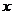,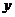,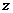are averages with length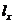,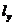and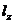respectively. The average for the whole sequence is represented bywith length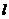, then the diagram can be read as the length weighted average decomposition as following relationship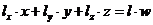(12)

< Previous | Next | Contents >